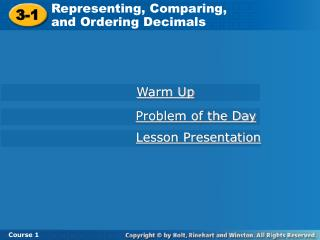DownloadDownload Presentation3-1

# 3-1

Télécharger la présentation## 3-1

- - - - - - - - - - - - - - - - - - - - - - - - - - - E N D - - - - - - - - - - - - - - - - - - - - - - - - - - -
##### Presentation Transcript

1. Representing, Comparing, and Ordering Decimals 3-1 Course 1 Warm Up Problem of the Day Lesson Presentation

2. Representing, Comparing, and Ordering Decimals 3-1 Course 1 Warm Up Order the numbers from least to greatest. 1.242, 156, 224, 165 2. 941, 148, 914, 814, 721 3. 345, 376, 354, 397 156, 165, 224, 242 148, 721, 814, 914, 941 345, 354, 376, 397

3. Representing, Comparing, and Ordering Decimals 3-1 Course 1 Problem of the Day Lupe is taller than Reba and shorter than Miguel. Tory is shorter than Lupe but taller than Reba. List the four brothers and sisters in order from tallest to shortest. Miguel, Lupe, Tory, Reba

4. Representing, Comparing, and Ordering Decimals 3-1 Course 1 Learn to write, compare, and order decimals using place value and number lines.

5. Representing, Comparing, and Ordering Decimals 3-1 Expanded form: Word form: Standard form: Word form: Course 1 Additional Example 1A & B: Reading and Writing Decimals Write each decimal in standard form, expanded form, and words. A. 1.07 B. 0.03 + 0.006 + 0.0009 1 + 0.07 one and seven hundredths 0.0369 three hundred sixty-nine ten-thousandths

6. Representing, Comparing, and Ordering Decimals 3-1 Standard form: Expanded form: Course 1 Additional Example C: Reading and Writing Decimals Write the decimal in standard form and expanded form. C. fourteen and eight hundredths 14.08 10 + 4 + 0.08

7. Representing, Comparing, and Ordering Decimals 3-1 Expanded form: Word form: Standard form: Word form: Course 1 Try This: Example 1A & 1B Write each decimal in standard form, expanded form, and words. A. 1.12 B. 0.6 + 0.008 + 0.0007 1 + 0.12 one and twelve hundredths 0.6087 six thousand eighty-seven ten-thousandths

8. Representing, Comparing, and Ordering Decimals 3-1 Standard form: Expanded form: Course 1 Try This: Example 1C Write each decimal in standard form and expanded form. C. eleven and two hundredths 11.02 10 + 1 + 0.02

9. Representing, Comparing, and Ordering Decimals 3-1 Line up the decimal points. Start from the left and compare the digits. Look for the first place where the digits are different. Course 1 Additional Example 2: Earth Science Application The star Wolf 359 has an apparent magnitude of 13.5. Suppose another star has an apparent magnitude of 13.05. Which star has the smaller magnitude? 13.50 13.05 0 is less than 5. 13.05 < 13.50 The star that has an apparent magnitude of 13.05 has the smaller magnitude.

10. Representing, Comparing, and Ordering Decimals 3-1 Line up the decimal points. Start from the left and compare the digits. Look for the first place where the digits are different. Course 1 Try This: Example 2 Tina reported on a star for her science project that has a magnitude of 11.3. Maven reported on another star that has a magnitude of 11.03. Which star has the smaller magnitude? 11.30 11.03 0 is less than 3. 11.03 < 11.30 The star that has a magnitude of 11.03 has the smaller magnitude.

11. Representing, Comparing, and Ordering Decimals 3-1 Compare two of the numbers at a time. Write 16.6 as “16.60.” Start at the left and compare the digits. Look for the first place where the digits are different. Course 1 Additional Example 3: Comparing and Ordering Decimals Order the decimals from least to greatest. 16.67, 16.6, 16.07 16.67 16.60 16.60 < 16.67 16.67 16.07 16.07 < 16.67 16.60 16.07 16.07 < 16.60

12. Representing, Comparing, and Ordering Decimals 3-1 16 16.1 16.2 16.3 16.4 16.5 16.6 16.7 16.8 16.9 17 Course 1 Additional Example 3 Continued Graph the numbers on a number line. 16.6 16.67 16.07 The numbers are ordered when you read the number line from left to right. The numbers in order from least to greatest are 16.07, 16.6, and 16.67.

13. Representing, Comparing, and Ordering Decimals 3-1 Compare two of the numbers at a time. Write 12.4 as “12.40.” Start at the left and compare the digits. Look for the first place where the digits are different. Course 1 Try This: Example 3 Order the decimals from least to greatest. 12.42, 12.4, 12.02 12.42 12.40 12.40 < 12.42 12.42 12.02 12.02 < 12.42 12.40 12.02 12.02 < 12.40

14. Representing, Comparing, and Ordering Decimals 3-1 12 12.1 12.2 12.3 12.4 12.5 12.6 12.7 12.8 12.9 13 Course 1 Try This: Example 3 Continued Graph the numbers on a number line. 12.42 12.4 12.02 The numbers are ordered when you read the number line from left to right. The numbers in order from least to greatest are 12.02, 12.4, and 12.42.

15. Representing, Comparing, and Ordering Decimals 3-1 Course 1 Insert Lesson Title Here Lesson Quiz: Part 1 Write each in standard form, expanded form and words. 1. 8.0342 2. 18 + 0.3 + 0.006 3. eight and twelve hundredths 8 + 0.03 + 0.004 + 0.0002; eight and three hundred forty-two ten thousandths 18.306; eighteen and three hundred six thousandths 8.12; 8 + 0.1 + 0.02

16. Representing, Comparing, and Ordering Decimals 3-1 Course 1 Insert Lesson Title Here Lesson Quiz: Part 2 4. It takes Pluto 246.7 years to orbit the Sun, and it takes Neptune 164.8 years. Which planet takes longer to orbit the Sun? 5. Order the decimals from least to greatest: 16.35, 16.3, 16.5. Pluto 16.3, 16.35, 16.5